A cache by Jaleco
Hidden : 09/03/2019
Difficulty: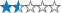Terrain: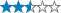Size: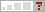(other)

#### Watch

How Geocaching WorksPlease note Use of geocaching.com services is subject to the terms and conditions in our disclaimer.

## Geocache Description:Vibrações Mecânicas Qualquer movimento que se repita após um determinado intervalo de tempo é chamado de vibração ou oscilação. Um sistema vibratório, em geral, inclui um meio para armazenar a energia potencial (mola ou elasticidade - k), um meio para armazenar energia cinética (massa ou inércia - m), e um meio a partir do qual a energia é dissipada (amortecedor - c). Na FIGURE 1, pode-se observar um modelo matemático discretizado (à direita) do modelo físico de uma mota (à esquerda). A Vibração Transversa de uma Corda ou Cabo Em muitos casos, conhecidos como sistemas contínuos ou distribuidos, não é possível identificar massas, amortecedores ou molas discretas. Devemos, então, considerar a distribuição contínua da massa, do amortecedor e da elasticidade e assumir que cada um dos infinitos pontos do sistema podem vibrar. Ver FIGURE 2. Se o sistema é modelado como discreto, the equações governantes são equações diferenciais ordinárias, que são relativamente fáceis de resolver. Por outro lado, se o sistema é modelado como contínuo, as equações governantes são equações diferenciais parciais, que são mais difíceis de resolver. A equação geral que rege a vibração de um sistema contínuo é a seguinte: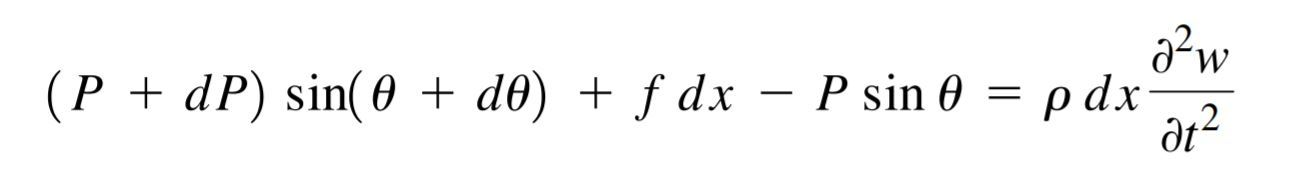A solução para o deslocamento transversal da corda que podes encontrar nas coordenadas da geocache (que tem as duas extremidades fixas) é a mostrada em baixo.O intuito desta geocache é dar a conhecer esta área de estudo fascinante e, quem sabe, despertar o teu interesse na mesma. Como é óbvio, a informação contida na listing está ultra condensada, pelo que se quiseres aprender mais, consulta, por exemplo: SINGIRESU, S. Rao, et al. Mechanical vibrations. Addison Wesley, 1995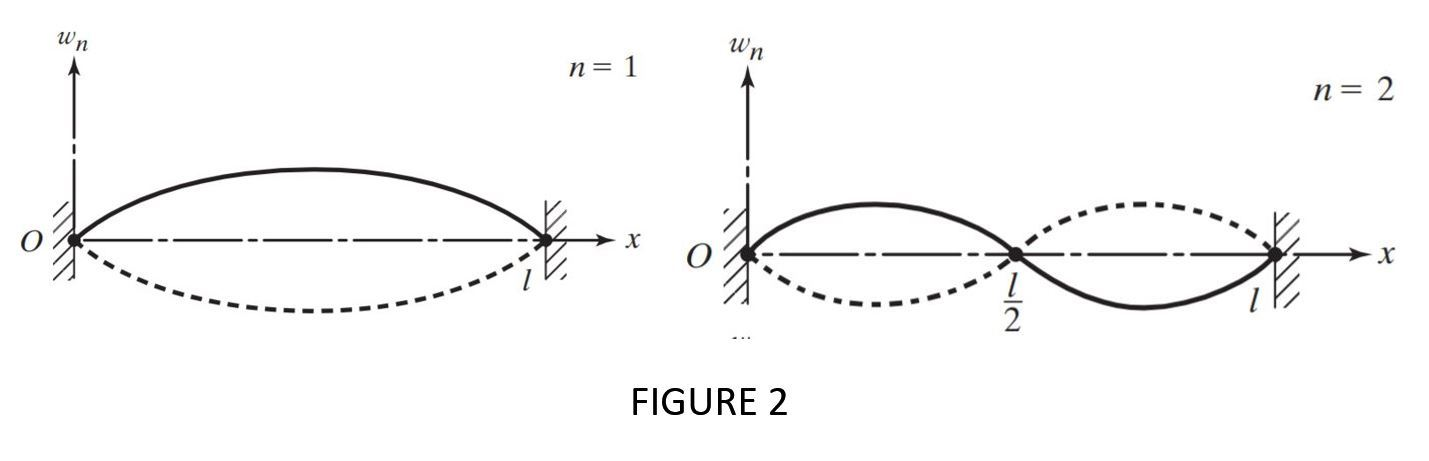Mechanical Vibrations Any motion that repeats itself after an interval of time is called vibration or oscillation. A vibratory system, in general, includes a means for storing potential energy (spring or elasticity - k), a means for storing kinetic energy (mass or inertia - m), and a means by which energy is gradually lost (damper - c). On FIGURE 1, you can see an exemple of a discrete mathematical model (on the right) for the physical sistem motorbike (on the left). The transverse Vibration of a String or Cable In many cases, known as distributed or continuous systems, it is not possible to identify discrete masses, dampers, or springs. We must then consider the continuous distribution of the mass, damping, and elasticity and assume that each of the infinite number of points of the system can vibrate. See FIGURE 2. If a system is modeled as a discrete one, the governing equations are ordinary differential equations, which are relatively easy to solve. On the other hand, if the system is modeled as a continuous one, the governing equations are partial differential equations, which are more difficult. The general equation which governs the vibration of a continuous system is:The solution of the String you can find at the Ground Zero (which has both ends fixed) can be expressed as shown bellow.The objective of this geocache is to make this fascinating field of study known, and, who knows, make you interested on it. Of course, the information contained in the listing are very incomplete, so if you want to learn more, check, for example: SINGIRESU, S. Rao, et al. Mechanical vibrations. Addison Wesley, 1995